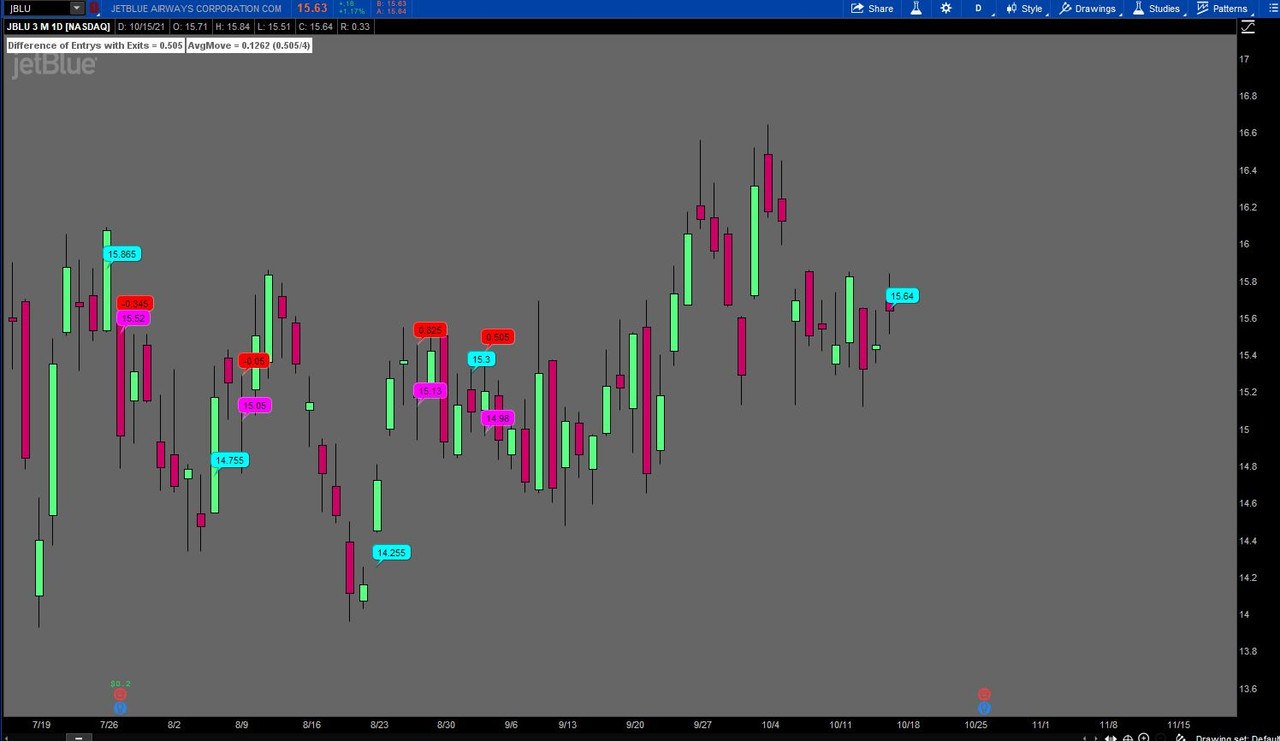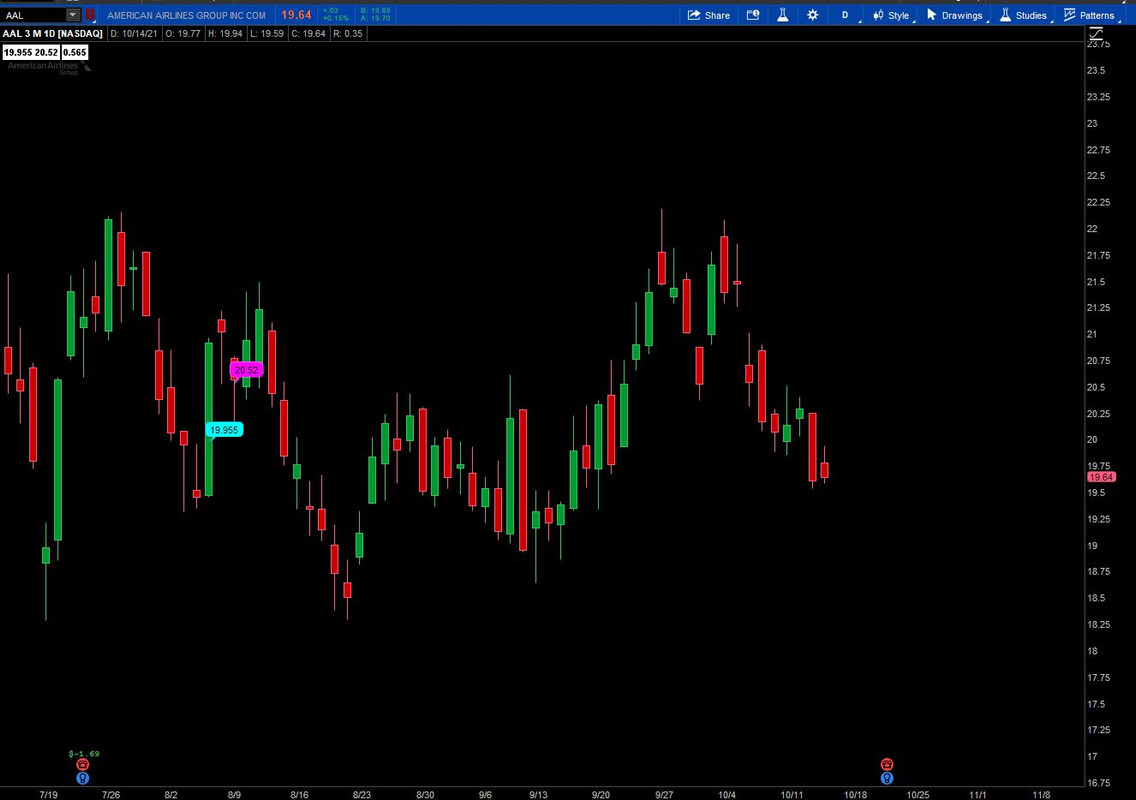# Having an issue with Incorrect code for count

#### greco26

##### Active member
Hi All,

Was wondering if someone can take a look at my code and tell me why my 'movediff' line isn't counting the difference between the exit and entries (the chart bubbles). My goal is to combine the historical difference in the entry and exits then divide by the number of times the trade triggered so that I can get the average move per trade. I've been at this all day and im stumped....

Code:
``````def h = high;
def l = low;
def o = open;
def c = close;

def insidebar =  (H <= H and L >= L);
def twodown  = H <= H and L < L;
def Cross_Up =  H > H;
def Mag_UP = H >= H;

# Strat Combos

def Two_One_UP =  twodown and insidebar and Cross_Up ;
def bn = barnumber();
def trigger =  Two_One_UP;
def triggercount = if trigger then triggercount + 1 else triggercount;
def on = if trigger then on * 0 + 1 else if on ==1 and low <low then on-1 else on;
def entry = if on == 1 and on == 0 then high else double.nan;
def exit = if on == 1 and on == 0 then low else double.nan;
def entryprice = if trigger and !exit then high else entryprice;
def exitprice = if exit then low else exitprice;

def movediff = if on ==1 and on ==0 then movediff + exitprice-entryprice else movediff;

def avgmovediff = movediff/triggercount;

Solution
Thanks for the response. I think the issue though is not the labels but rather the line of code that should be counting the difference between entry and exit. I have the labels just to visualize.

Ok, here is a revision to show the movediff based upon entrys and exits only when there is an exit after an entry. Any entry without an exit will not be counted until there is an exit. A bubble is now above each exit to show the running total difference of exitprice - entryprice. Triggercount was revised to only count exits.Ruby:
``````def h = high;
def l = low;
def o = open;
def c = close;

def insidebar =  (h <= h and l >= l);
def twodown  = h <= h and l < l;
def Cross_Up =  h > h;
def Mag_UP = h >= h;

#...``````
Hi All,

Was wondering if someone can take a look at my code and tell me why my 'movediff' line isn't counting the difference between the exit and entries (the chart bubbles). My goal is to combine the historical difference in the entry and exits then divide by the number of times the trade triggered so that I can get the average move per trade. I've been at this all day and im stumped....

Code:
``````def h = high;
def l = low;
def o = open;
def c = close;

def insidebar =  (H <= H and L >= L);
def twodown  = H <= H and L < L;
def Cross_Up =  H > H;
def Mag_UP = H >= H;

# Strat Combos

def Two_One_UP =  twodown and insidebar and Cross_Up ;
def bn = barnumber();
def trigger =  Two_One_UP;
def triggercount = if trigger then triggercount + 1 else triggercount;
def on = if trigger then on * 0 + 1 else if on ==1 and low <low then on-1 else on;
def entry = if on == 1 and on == 0 then high else double.nan;
def exit = if on == 1 and on == 0 then low else double.nan;
def entryprice = if trigger and !exit then high else entryprice;
def exitprice = if exit then low else exitprice;

def movediff = if on ==1 and on ==0 then movediff + exitprice-entryprice else movediff;

def avgmovediff = movediff/triggercount;

These were values rather than true/false (1,0)

def entry = if on == 1 and on == 0 then 1 else 0;
def exit = if on == 1 and on == 0 then 1 else 0;

Here is revised code with a 3moD chart of AAL with one set of bubbles that now match the labels shown.Ruby:
``````def h = high;
def l = low;
def o = open;
def c = close;

def insidebar =  (H <= H and L >= L);
def twodown  = H <= H and L < L;
def Cross_Up =  H > H;
def Mag_UP = H >= H;

# Strat Combos

def Two_One_UP =  twodown and insidebar and Cross_Up ;
def bn = barnumber();
def trigger =  Two_One_UP;
def triggercount = if trigger then triggercount + 1 else triggercount;
def on = if trigger then on * 0 + 1 else if on ==1 and low <low then on-1 else on;
def entry = if on == 1 and on == 0 then 1 else 0;
def exit = if on == 1 and on == 0 then 1 else 0;
def entryprice = if trigger and !exit then high else entryprice;
def exitprice = if exit then low else exitprice;
addlabel(1, entryprice + " "+ exitprice, color.white);
def movediff = if on ==1 and on ==0 then movediff + exitprice-entryprice else movediff;

def avgmovediff = movediff/triggercount;

•greco26
These were values rather than true/false (1,0)

def entry = if on == 1 and on == 0 then 1 else 0;
def exit = if on == 1 and on == 0 then 1 else 0;

Here is revised code with a 3moD chart of AAL with one set of bubbles that now match the labels shown.
Thanks for the response. I think the issue though is not the labels but rather the line of code that should be counting the difference between entry and exit. I have the labels just to visualize.

Thanks for the response. I think the issue though is not the labels but rather the line of code that should be counting the difference between entry and exit. I have the labels just to visualize.

Ok, here is a revision to show the movediff based upon entrys and exits only when there is an exit after an entry. Any entry without an exit will not be counted until there is an exit. A bubble is now above each exit to show the running total difference of exitprice - entryprice. Triggercount was revised to only count exits.Ruby:
``````def h = high;
def l = low;
def o = open;
def c = close;

def insidebar =  (h <= h and l >= l);
def twodown  = h <= h and l < l;
def Cross_Up =  h > h;
def Mag_UP = h >= h;

# Strat Combos

def Two_One_UP =  twodown and insidebar and Cross_Up ;
def bn = BarNumber();
def trigger =  Two_One_UP;

def on = if trigger then on * 0 + 1 else if on == 1 and low < low then on - 1 else on;
def entry = if on == 1 and on == 0 then 1 else 0;
def exit = if on == 1 and on == 0 then 1 else 0;
def entryprice = if trigger and !exit then high else entryprice;
def exitprice = if exit then low else exitprice;
def moveentry = if entry then moveentry + entryprice else moveentry;
def moveexit = if exit then moveexit + exitprice else moveexit;
def movediff = if  exit then movediff + (exitprice - entryprice) else movediff;
def triggercount = if exit then triggercount + 1 else triggercount;
def avgmovediff = movediff / triggercount;

AddLabel(yes, "Difference of Entrys with Exits = " + movediff, Color.WHITE);
AddLabel(1, "AvgMove = " + avgmovediff + " (" + movediff + "/" + triggercount + ")", Color.WHITE);

### Not the exact question you're looking for?

87k+ Posts
292 Online## The Market Trading Game Changer

Join 2,500+ subscribers inside the useThinkScript VIP Membership Club
• Exclusive indicators
• Proven strategies & setups
• Private Discord community
• Exclusive members-only content
• 1 full year of unlimited support

What is useThinkScript?

useThinkScript is the #1 community of stock market investors using indicators and other tools to power their trading strategies. Traders of all skill levels use our forums to learn about scripting and indicators, help each other, and discover new ways to gain an edge in the markets.

How do I get started?

We get it. Our forum can be intimidating, if not overwhelming. With thousands of topics, tens of thousands of posts, our community has created an incredibly deep knowledge base for stock traders. No one can ever exhaust every resource provided on our site.

If you are new, or just looking for guidance, here are some helpful links to get you started.

What are the benefits of VIP Membership?